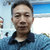# 高一化学----氧化还原反应导学案

• 10人评价）
• 897人阅读
• 上传者：祁永花

### 相关文档推荐

2018版化学（苏教版）高考总复习对接高考精练--氧化还原反应基础

2018年高考化学总复习 课时跟踪检测 五　氧化还原反应

《氧化还原反应》周测试题

2016年高考化学真题分类汇编 专题04 氧化还原反应 Word版含解析[www.ks5u.com 高考]

2015年人教版 必修一 第二章 第三节氧化还原反应基础巩固练习

【思远 行远】 新人教版必修1 第二章 化学物质及其变化 第三节　氧化还原反应 02

【思远 行远】2016版高二化学暑假作业—— 专题10 氧化还原反应的规律0积分 下载
```                 中国现代教育网      www.30edu.com  全国最大教师交流平台

氧化还原反应 学案

(1) CaO + H2O = Ca(OH)2

(2) 4Fe + 3O2 = 2Fe2O3

(3) CaCl2 + Na2CO3 = CaCO3 + 2NaCl

(4) H2CO3 = CO2 + H2O

(5) CuO + H2 = Cu +H2O

(6) 2NaHCO3 = Na2CO3 + H2O + CO2

(7) 2Mg + O2 = 2MgO

(8) NaCl + AgNO3 = AgCl + NaNO3

(9) 3CO + Fe2O3 = 3CO2 + 2Fe

(10) Cu + 2H2SO4 = CuSO4 + SO2 + 2H2O

分类标准     1：________________分类标准         2：
________________

第一类反应可用“A”表示；第二类反应可用“B”表

(1) CaO + H2O = Ca(OH)2

(2) 4Fe + 3O2 = 2Fe2O3

(3) CaCl2 + Na2CO3 = CaCO3 + 2NaCl

(4) H2CO3 = CO2 + H2O

(5) CuO + H2 = Cu +H2O

(6) 2NaHCO3 = Na2CO3 + H2O + CO2

(7) 2Mg + O2 = 2MgO

(8) NaCl + AgNO3 = AgCl + NaNO3

(9) 3CO + Fe2O3 = 3CO2 + 2Fe

(10) Cu + 2H2SO4 = CuSO4 + SO2 + 2H2O
中国现代教育网    www.30edu.com  全国最大教师交流平台

氧化铁炼铁                               铁生锈

3CO + Fe2O3 = 3CO2 + 2Fe                   4Fe + 3O2 = 2Fe2O3

(1)2Na + Cl2 = 2NaCl

(2)NaOH + HCl = NaCl + H2O

(3)Zn+CuSO4 = ZnSO4 + Cu

2Na + Cl2 = 2NaCl

(1)产物中的微粒是什么？

(2)反应物中的微粒是什么？

(3)他们是如何转变的？

0积分下载